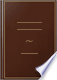Søk Bilder Maps Play YouTube Nyheter Gmail Disk Mer »
Logg på
 Bøker Bok 61–70 av 161 på A rhomboid, is that which has its opposite sides equal to one another, but all its...A rhomboid, is that which has its opposite sides equal to one another, but all its sides are not equal, nor its angles right angles.The Elements of Euclid: Viz. the First Six Books, Together with the Eleventh ... - Side 2
av Euclides - 1816 - 528 sider
Uten tilgangsbegrensning - Om denne bokenThe Elements of Geometry, Symbolically Arranged

1846
...four-sided figures, a square is that which has all its angles right angles, and all its sides equal. XXXI. An oblong is that which has all its angles right...its sides equal. XXXII. A rhombus is that which has all its sides equal, but its angles are not right angles. XXXIII. A rhomboid is that which has its...
Uten tilgangsbegrensning - Om denne bokenThe Elements of Geometry, Symbolically Arranged

1846
...four-sided figures, a square is that which has all its angles right angles, and all its sides equal. XXXI. An oblong is that which has all its angles right angles, but has not all its sides equal. XXXII. XXXIII. A rhomboid is that which has its opposite sides equal to one / another, but all its sides are...
Uten tilgangsbegrensning - Om denne bokenA Course of Practical Geometry for mechanics

William Pease - 1846
...will necessarily be acute. 54*. An Acute-angled Triangle is that which has three acute angles. 55*. Of four-sided figures, a square is that which has...its sides equal, and all its angles right angles. 56*. An oblong is that which has all its angles right angles, but has not all its sides equal. Its...
Uten tilgangsbegrensning - Om denne bokenElements of Geometry: Containing the First Six Books of Euclid, with a ...

John Playfair - 1846 - 317 sider
...obtuse angle. 24. An acute angled triangle is that which has three acute angles. 25. Of four sided figures, a square is that which has all its sides equal and all its angles right angles. 26. An oblong is that which has all its angles right angles, but has not all its sides equal. 28. A...
Uten tilgangsbegrensning - Om denne bokenElements of Drawing and Mensuration Applied to the Mechanic Arts: A Book for ...

Charles Davies - 1846 - 240 sider
...altitude. Thus, AC is the altitude. 9. Name the different kinds of quadrilaterals. First. — The square, which has all its sides equal, and all its angles right angles. Second. — The rectangle, which has its angles right angles, and its opposite sides equal and parallel....
Uten tilgangsbegrensning - Om denne bokenThe first book of Euclid's Elements, simplified, explained and illustrated ...

Euclides - 1847
...that which has an obtuse angle. 28. An acute.angled triangle is that which has three acute angles. 29. Of four.sided figures, a square is that which has...its sides equal, and all its angles right angles. 30. An oblong is that which has all its angles right angles, but has not all its sides equal. Called...
Uten tilgangsbegrensning - Om denne bokenFirst Lessons in Geometry: Upon the Model of Colburn's First Lessons in ...

Alpheus Crosby - 1847 - 164 sider
...before any demonstration of the properties of quadrilaterals, the square is defined as "a quadrilateral, which has all its sides equal, and all its angles right angles " ; the rectangle, as one " which has its opposite sides equal, and its angles right angles " ; the...
Uten tilgangsbegrensning - Om denne bokenAn Essay Upon the Study of Geometry in Common Schools

Stephen Chase - 1847 - 26 sider
...before any demonstration of the properties of quadrilaterals, the square is defined as "a quadrilateral, which has all its sides equal, and all its angles right angles " ; the rectangle, as one " which has its opposite sides equal, and its angles right angles " ; the...
Uten tilgangsbegrensning - Om denne bokenThe definitions, postulates, axioms, and enunciations of the propositions of ...

Euclides - 1848
...triangle is that which has an obtuse angle. XXIX. XXX. Of quadrilateral or four-sided figures, a square has all its sides equal and all its angles right angles....but has not all its sides equal. XXXII. A rhombus has all its sides equal, but its angles are not right angles. XXXIII. A rhomboid has its opposite sides...
Uten tilgangsbegrensning - Om denne bokenElements of Geometry, Theoretical and Practical: Containing a Full ...

George Clinton Whitlock - 1848 - 324 sider
...21°. Of all spherical polygons having the same number of sides and the same perimeter, the greatest is that which has all its sides equal and all its angles equal. 22°. Spherical pyramids, which stand upon equal bases, are equal to one another ; so, likewise,...
Uten tilgangsbegrensning - Om denne boken